Next: Conclusions Up: Scalable Data Parallel Algorithms Previous: Maximum Likelihood Estimate

# Texture Compression

We implement an algorithm for compressing an image of a GMRF texture to approximately 1 bit/pixel from the original 8 bits/pixel image. The procedure is to find the MLE of the given image, (e.g. this results in a total of eleven 32-bit floating point numbers for the 4th order model). We then use a Max Quantizer, with characteristics given in , to quantize the residual to 1-bit. The quantized structure has a total of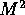bits. To reconstruct the image from its texture parameters and 1-bit Max quantization, we use an algorithm similar to Algorithm 2. Instead of synthesizing a texture from Gaussian noise, we begin with the 1-bit quantized array. Compressed textures for a 4th order model are shown in Figures 6 and 7. A result using the higher order model is shown in Figure 8.

The noise sequence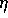is generated as follows: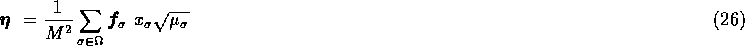where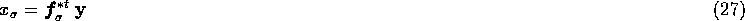and the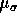is given in (13). We estimate the residual as: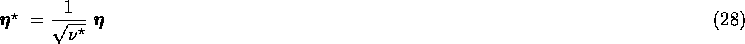and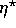is the sequence which is Max quantized.

The image reconstruction from parameters and quantizationis as follows: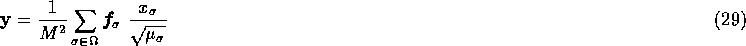where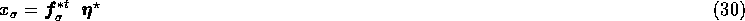andis given in (13);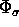is given in (14).

The texture compression algorithm has the same time complexity and scalability characteristics as Algorithm 4. The image reconstruction algorithm has the same complexities as Algorithm 2. Hence these algorithms scale with image size n and number of processors p.

This algorithm could be used to compress the textures regions in natural images as part of segmentation based compression schemes discussed in . Compression factors of 35 have been obtained for the standard Lena and F-16 images, with no visible degradations. Compression factors of 80 have been shown to be feasible when small degradations are permitted in image reconstruction.Next: Conclusions Up: Scalable Data Parallel Algorithms Previous: Maximum Likelihood Estimate